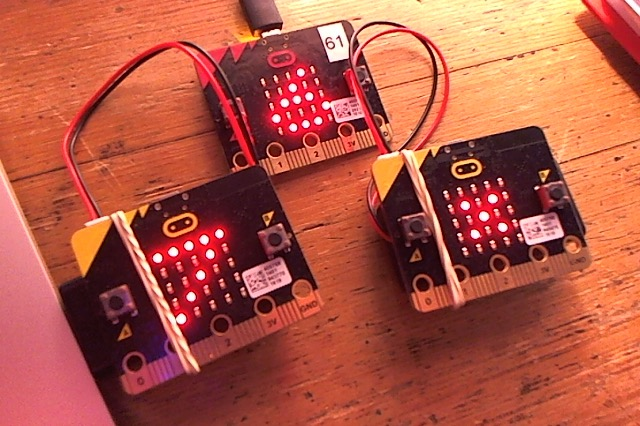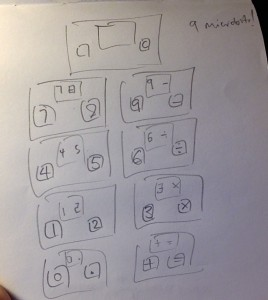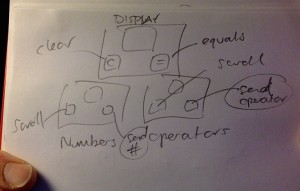# Making calculators with microbitsWell, making a calculator with THREE micro:bits really. This is, I admit, a bit of a mad project but I’ve been fascinated by calculators since childhood. I can remember cheap pocket calculators being invented and, like digital watches, I still think they are a pretty neat idea.Inspired by Philip Meitiner’s keypad micro:bit calculator, I wondered if I could make one just using micro:bits. My first sketch used NINE micro:bits in a grid, which I then decided was utterly bonkers, so I cut it down to three (though you could do this project with two, but I like three. It’s the magic number, don’t you know?)

This was quite a pleasant Easter Sunday intellectual diversion, and I have a new-found respect for designers of humble adding machines: it’s harder than it looks.

Here’s how it works. The top micro:bit is the display which shows entered numbers and operators. The left button A is the clear button. The right button B is the ‘=’ button (equals, calculate, evaluate, work it out!)Underneath it are two more micro:bits. The one on the left does numbers, the one on the right sends operators. Using the A button, you scroll through each possible number (0 through to 9 and . for decimal point) and operators (+,-,/ and *). When you found have the number you want to send, you press button B to transmit it over radio to the display. Press button B on the display micro:bit to calculate the result – if it’s longer than a single digit it scrolls, but you can press button B again if you missed it.

I had to think quite hard about how a calculator’s UI works and how it knows when to perform the actual calculation. My solution doesn’t emulate a real calculator – it doesn’t have a keypad for one thing. It builds up a string of numbers and operations until you press the = button, then it evaluates the string. If there’s no operator, pressing = does nothing. If there is no second number, it does nothing. If there is an operator in the string and 2 numbers it will perform the calculation.

I’m sure the code can be made more elegant but I’m quite pleased with it. It copes with decimal numbers, if you don’t have an operator or a second number it doesn’t error but waits for you to add them. Perhaps you could add more mathematical functions? Error handling for more than 2 numbers or division by zero?

Here’s a short video showing how it works in practice:

This is the program you flash to the number encoder:

```from microbit import *
numbers = ["0","1","2","3","4","5","6","7","8","9","."]
x = 0

while True:
if button_a.was_pressed():
x += 1
if x > 10:
x = 0
display.show(numbers[x])
if button_b.was_pressed():
```

This is the program you flash to the operator encoder:

```from microbit import *
operators = ["+","-","/","*"]

x = 0

while True:
if button_a.was_pressed():
x += 1
if x > 3:
x = 0
display.show(operators[x])
if button_b.was_pressed():

And finally, this is the program you flash to the display micro:bit:

```from microbit import *
calc_string = ""

def displayResult():
result_string = str(result)
if result_string[-2:] == ".0":    # strip trailing .0 from whole numbers
result_string = result_string[:-2]
if len(result_string) == 1:
display.show(result_string)   # show single digits
else:
display.scroll(result_string) # scroll longer numbers

while True:
if incoming:
display.show(incoming)
calc_string = calc_string + incoming
if button_a.was_pressed():   # clear button
calc_string = ""
a = None
b = None    # destroy b so you can test it exists later
display.show("0")
if button_b.was_pressed():   # equals button
if "+" in calc_string or "-" in calc_string or "/" in calc_string or "*" in calc_string:
z = 0
for char in calc_string:
if char in "+-/*":
operator = char
a = calc_string[:z]   # a is everthything before the operator
b = calc_string[z+1:] # b is everything after the operator
z += 1
if operator == "+" and b: # check 2nd number exists before displaying result
result = float(a) + float(b)
displayResult()
if operator == "-" and b:
result = float(a) - float(b)
displayResult()
if operator == "*" and b:
result = float(a) * float(b)
displayResult()
if operator == "/" and b:
result = float(a) / float(b)
displayResult()
else:
print("no operator")
```
This entry was posted in computers, microbit and tagged , , . Bookmark the permalink.